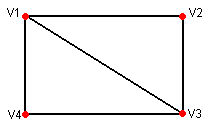# How to assign UV coordinates to arbitrary mesh.

I have a wall that is randomly generated.And I need to assign a UV to it. I need any texture I apply to have a constant tile across the wall. Am I going to have to work out magnitudes between vertexes? What’s the easiest way to accomplish this?

If you just want to have a constant 1:1 aspect along each segment, things are easier. If you have to ensure continuity from one segment to the next, then you must also set the uv offset and keep track of the current position when generating new segments.

Supposing that you have just calculated the four vertex of a new segment in variables v1 to v4, where v1 is the top-left vertex, v2 is top-right, v3 is bottom-right and v4 is bottom-left, their corresponding uv coordinates can be calculated in variables uv1 to uv4 as follows:``````  ...
var segW = Vector3.Distance(v1, v2); // calculate segment width
var segH = Vector3.Distance(v1, v4); // calculate segment height
var rightX: float = segW/segH; // calculate right X coordinate
uv1 = Vector2(0,1); // uv relative to the top-left vertex
uv2 = Vector2(rightX,1); // uv relative to the top-right vertex
uv3 = Vector2(rightX,0); // uv relative to the bottom-right vertex
uv4 = Vector2(0,0); // uv relative to the bottom-left vertex
...
``````

If you need continuity, then a curX member variable must be kept and updated after generating each new segment:

``````var curX: float = 0; // define curX outside any function

...
var segW = Vector3.Distance(v1, v2); // calculate segment width
var segH = Vector3.Distance(v1, v4); // calculate segment height
var rightX: float = curX + segW/segH; // calculate right X coord
uv1 = Vector2(curX,1); // uv relative to the top-left vertex
uv2 = Vector2(rightX,1); // uv relative to the top-right vertex
uv3 = Vector2(rightX,0); // uv relative to the bottom-right vertex
uv4 = Vector2(curX, 0); // uv relative to the bottom-left vertex
// update curX to the next wall segment. Use only the fractionary
// part, since big uv values may cause visible artifacts due to
// the limited precision used in shaders
curX = rightX % 1;
...
``````